Next: Diagonals of a parallelogram Up: Motion in 3 dimensions Previous: Vector magnitude

## Scalar multiplication

Suppose that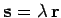. This expression is interpreted as follows: vector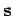points in the same direction as vector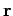, but the length of the former vector is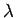times that of the latter. Note that ifis negative then vectorpoints in the opposite direction to vector, and the length of the former vector is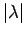times that of the latter. In terms of components: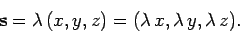(37)

In other words, when we multiply a vector by a scalar then the components of the resultant vector are obtained by multiplying all the components of the original vector by the scalar.

Richard Fitzpatrick 2006-02-02